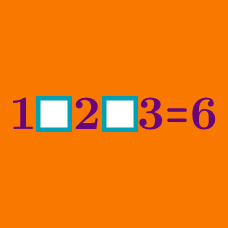Logic

# Operator Search: Level 3 Challenges

$1 \ \boxed{\phantom{0}} \ 2 \ \boxed{\phantom{0}} \ 3 \ \boxed{\phantom{0}} \ 4 \ \boxed{\phantom{0}} \ 5$

Use the mathematical operators $+,-,÷,×$ exactly once to fill in the blank boxes. What is the maximum real value that can be obtained?

Using only the 4 basic arithmetic operations ($+, -, \times, \div$), what is the largest possible value of

$\large 1\, \square\, 2\,\square \,3\, \square \, 4 ?$

$\large 8 \ \Box \ 8 \ \Box \ 8 \ \Box \ 8 \ \Box \ 8 \ \Box \ 8\ \Box \ 8 \ \Box\ 8=1000$

What is the minimum number of operators that can be filled in the boxes to make the equation above true?

Note: Boxes can be left blank to denote concetanation of adjacent digits

\large{\begin{aligned} 1 &=& 44 \div 44 \\ 2 &=& 4 \times 4 \div (4 + 4 ) \\ 3 &=& (4 + 4 + 4) \div 4 \\ 4 &=& 4 + (4\times(4-4)) \\ 5 &=& (4 + (4\times4)) \div 4 \end{aligned}}

Above shows the first 5 positive integers formed by using the four mathematical operators ($+ \ - \ \times \ \div$) only on the digit 4 four times.

What is the smallest positive integer that cannot be represented using these conditions?

Note: You are allowed to join the digits together: $44 + 44$.

$\large 1 \; \underbrace{\square \; 2 \; \square \; \cdots \; \square \; n \; \square}_{n \text{ number of }\square\text{'s}} \; (n+1) = n+ 2$

What is the minimum value of the positive integer $n>1$ such that we can fill in all the boxes above by using at least one of the four mathematical operators ( $+, -, \times , \div$ ) and the equation holds true?

Note: Order of operations (BODMAS) applied.

×